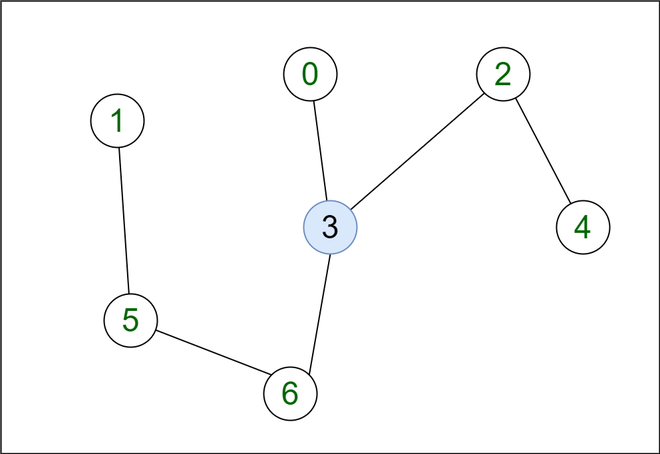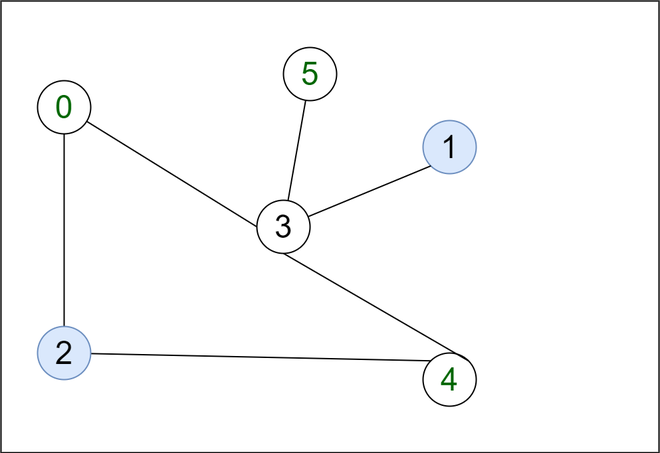# Count of connected components in given graph after removal of given Q vertices

• Last Updated : 25 Mar, 2022

Given an undirected graph g, the task is to find the number of coalitions formed in it after the removal of Q vertices and maximum vertices among all these connected components.

A coalition is defined as the number of connected components left after removal of Q vertices i.e vertices being removed from the graph are not considered as part of the coalition.

Example:

Input: V = 7, E = 6, Q = 1

Edges: { {0, 3}, {1, 5}, {3, 6}, {3, 2}, {2, 4}, {5, 6} }

queries: {3}Output: 3 3
Explanation:

If we remove vertices 3 from the graph then their corresponding edges {{0, 3}, {3, 6}, {3, 2}} will also be get removed from the graph and the number of the connected components excluding Q (removed vertices) are {0}. {1, 5, 6} and {2, 4} with maximum coalition of {1, 5, 6} as 3.

Input: V = 6, E = 6, Q = 2

Edges: {{0, 3}, {0, 2}, {3, 4}, {3, 5}, {3, 1}, {2, 4}}

queries: {1, 2}Output: 1 4
Explanation: When vertex 1 and 2 is removed from the given graph the connected component excluding Q is {0, 3, 4, 5} with maximum coalition of {0, 3, 4, 5} as 4.

Approach: The approach to solve this problem based on following idea

The idea here is, if we remove all unnecessary edges that connects with the vertex mentioned in the query, then the problem will break down to find the number of connected component in undirected graph.

Based on above idea follow the steps below to implement this approach:

1. We can use the multimap to store edges between the vertices.
2. We remove all edges that are connected with the vertices (because we assume that we will have to delete all the vertices from the Query then, their corresponding edges should also be get remove that are connected with the vertices)
3. Find the number of connected component in undirected graph.
• Use a variable count to store the number of connected components, cnt to keep count of number of vertices of the component we are traversing and maxLen to store maximum vertices among all components ( maximum of cnt ).
4. Calculate coalitions = count ( number of connected components ) – size of query ( number of vertices removed)

Below is the implementation of the above approach:

## C++14

 `#include ``using` `namespace` `std;``int` `maxLen = 0;``int` `cnt;` `class` `Graph {``    ``int` `V;` `    ``list<``int``>* adj;``    ``multimap<``int``, ``int``> adj2;``    ``void` `DFSUtil(``int` `v, ``bool` `visited[]);` `public``:``    ``Graph(``int` `V);` `    ``void` `addEdges();``    ``void` `removeEdges(``int` `V, ``int` `Edges[],``                     ``int` `E, ``int` `queries[],``                     ``int` `Q);``    ``int` `NumberOfconnectedComponents();``};` `// function to find the number of connected``// component in undirected graph``int` `Graph::NumberOfconnectedComponents()``{` `    ``bool``* visited = ``new` `bool``[V];` `    ``int` `count = 0;``    ``for` `(``int` `v = 0; v < V; v++)``        ``visited[v] = ``false``;` `    ``for` `(``int` `v = 0; v < V; v++) {``        ``if` `(visited[v] == ``false``) {``            ``cnt = 0;``            ``DFSUtil(v, visited);``            ``count += 1;``        ``}``    ``}` `    ``return` `count;``}` `void` `Graph::DFSUtil(``int` `v, ``bool` `visited[])``{``    ``visited[v] = ``true``;``    ``cnt++;` `    ``list<``int``>::iterator i;` `    ``for` `(i = adj[v].begin(); i != adj[v].end(); ++i)``        ``if` `(!visited[*i]) {``            ``DFSUtil(*i, visited);``        ``}``    ``maxLen = max(maxLen, cnt);``}` `Graph::Graph(``int` `V)``{``    ``this``->V = V;``    ``adj = ``new` `list<``int``>[V];``}` `// add Edges in the graph``void` `Graph::addEdges()``{``    ``for` `(``auto` `x : adj2) {``        ``adj[x.first].push_back(x.second);``        ``adj[x.second].push_back(x.first);``    ``}``}` `// function to remove all the edges that are``// connected with vertices``void` `Graph::removeEdges(``int` `V, ``int` `Edges[],``                        ``int` `E, ``int` `queries[],``                        ``int` `Q)``{``    ``multimap<``int``, ``int``> adj3;``    ``for` `(``int` `i = 0; i < E; i++)``        ``adj3.insert({ Edges[i], Edges[i] });` `    ``for` `(``int` `i = 0; i < Q; i++) {``        ``auto` `it1 = adj3.lower_bound(queries[i]);``        ``auto` `it2 = adj3.upper_bound(queries[i]);``        ``adj3.erase(it1, it2);``    ``}` `    ``for` `(``auto` `it = adj3.begin(); it != adj3.end(); it++) {``        ``adj2.insert({ it->second, it->first });``    ``}``    ``for` `(``int` `i = 0; i < Q; i++) {``        ``auto` `it1 = adj2.lower_bound(queries[i]);``        ``auto` `it2 = adj2.upper_bound(queries[i]);``        ``adj2.erase(it1, it2);``    ``}``}` `// Driver code``int` `main()``{``    ``int` `V = 7, E = 6;``    ``Graph g(V);``    ``int` `Edges[] = { { 0, 3 }, { 1, 5 }, { 3, 6 }, { 3, 2 }, { 2, 4 }, { 5, 6 } };``    ``int` `Q = 1;``    ``int` `queries[] = { 3 };``    ``// remove all the edges that are connected with``    ``// vertices given in the queries``    ``g.removeEdges(V, Edges, E, queries, Q);` `    ``g.addEdges();` `    ``cout << g.NumberOfconnectedComponents() - Q;``    ``cout << ``" "` `<< maxLen;` `    ``return` `0;``}`

Output

`3 3`

Time complexity: O(V + E*log(E)), where V is the number of vertices and E is the number of edges in the graph.
Auxiliary Space: O(V), since an extra visited array of size V is required.

My Personal Notes arrow_drop_up